The Australian Journal of Mathematical Analysis and Applications

 Home News Editors Volumes RGMIA Subscriptions Authors Contact

ISSN 1449-5910

You searched for qi
Total of 247 results found in site

27: Paper Source PDF document

Paper's Title:

Some Inequalities of the Hermite-Hadamard Type for k-Fractional Conformable Integrals

Author(s):

C.-J. Huang, G. Rahman, K. S. Nisar, A. Ghaffar and F. Qi

Department of Mathematics, Ganzhou Teachers College,
Ganzhou 341000, Jiangxi,
China.
E-mail: hcj73jx@126.com , huangcj1973@qq.com

Department of Mathematics, Shaheed Benazir Bhutto University,
Sheringal, Upper Dir, Khyber Pakhtoonkhwa,
Pakistan.
E-mail: gauhar55uom@gmail.com

Department of Mathematics, College of Arts and Science at Wadi Aldawaser, 11991,
Prince Sattam Bin Abdulaziz University, Riyadh Region,
Kingdom of Saudi Arabia.
E-mail: n.sooppy@psau.edu.sa, ksnisar1@gmail.com

Department of Mathematical Science, Balochistan University of Information Technology,
Engineering and Management Sciences, Quetta,
Pakistan.
E-mail: abdulghaffar.jaffar@gmail.com

School of Mathematical Sciences, Tianjin Polytechnic University,
Tianjin 300387,
China; Institute of Mathematics,
Henan Polytechnic University, Jiaozuo 454010, Henan,
China.
E-mail: qifeng618@gmail.com, qifeng618@qq.com

Abstract:

In the paper, the authors deal with generalized k-fractional conformable integrals, establish some inequalities of the Hermite-Hadamard type for generalized k-fractional conformable integrals for convex functions, and generalize known inequalities of the Hermite-Hadamard type for conformable fractional integrals.

23: Paper Source PDF document

Paper's Title:

On A Conjecture of A Logarithmically Completely Monotonic Function

Author(s):

Valmir Krasniqi, Armend Sh. Shabani

Department of Mathematics,
University of Prishtina,
Republic of Kosova

E-mail: vali.99@hotmail.com
armend_shabani@hotmail.com

Abstract:

In this short note we prove a conjecture, related to a logarithmically completely monotonic function, presented in . Then, we extend by proving a more generalized theorem. At the end we pose an open problem on a logarithmically completely monotonic function involving q-Digamma function.

22: Paper Source PDF document

Paper's Title:

Inequalities Relating to the Gamma Function

Author(s):

Chao-Ping Chen and Feng Qi

Department of Applied Mathematics and Informatics, Research Institute of Applied Mathematics,
Henan Polytechnic University, Jiaozuo City, Henan 454000, China

chenchaoping@hpu.edu.cn

Department of Applied Mathematics and Informatics, Research Institute of Applied Mathematics,
Henan Polytechnic University, Jiaozuo City, Henan 454000, China

qifeng@hpu.edu.cn, fengqi618@member.ams.org

U
rl: http://rgmia.vu.edu.au/qi.html, http://dami.hpu.edu.cn/qifeng.html

Abstract:

For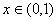, we have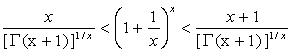.

For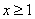,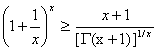,

And equality occurs for x=1.

18: Paper Source PDF document

Paper's Title:

A Double Inequality for Divided Differences and Some Identities of the Psi and Polygamma Functions

Author(s):

B. N. Guo and F. Qi

School of Mathematics and Informatics, Henan Polytechnic University, Jiaozuo City, Henan Province, 454010, China
bai.ni.guo@gmail.com, bai.ni.guo@hotmail.com

School of Research Institute of Mathematical Inequality Theory, Henan Polytechnic University, Jiaozuo City, Henan Province, 454010, China
qifeng618@gmail.com, qifeng618@hotmail.com, qifeng618@qq.com
URL:http://qifeng618.spaces.live.com

Abstract:

In this short note, from the logarithmically completely monotonic property of the function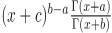, a double inequality for the divided differences and some identities of the psi and polygamma functions are presented.

14: Paper Source PDF document

Paper's Title:

A Wallis Type Inequality and a Double Inequality for Probability Integral

Author(s):

Jian Cao, Da-Wei Niu and Feng Qi

School of Mathematics and Informatics,
Henan Polytechnic University, Jiaozuo City,
Henan Province, 454010,
China
21caojian@163.com

School of Mathematics and Informatics,
Henan Polytechnic University, Jiaozuo City,
Henan Province, 454010,
China
nnddww@tom.com

Research Institute of Mathematical Inequality Theory,
Henan Polytechnic University, Jiaozuo City,
Henan Province, 454010,
China
qifeng@hpu.edu.cn
fengqi618@member.ams.org
qifeng618@hotmail.com
qifeng618@msn.com
qifeng618@qq.com
URL: http://rgmia.vu.edu.au/qi.html

Abstract:

In this short note, a Wallis type inequality with the best upper and lower bounds is established. As an application, a double inequality for the probability integral is found.

12: Paper Source PDF document

Paper's Title:

Monotonicity Properties for Generalized Logarithmic Means

Author(s):

Chao-Ping Chen and Feng Qi

Department of Applied Mathematics and Informatics, Research Institute of Applied Mathematics,
Henan Polytechnic University, Jiaozuo City, Henan 454000, China

chenchaoping@hpu.edu.cn

Department of Applied Mathematics and Informatics, Research Institute of Applied Mathematics,
Henan Polytechnic University, Jiaozuo City, Henan 454000, China

qifeng@hpu.edu.cn, fengqi618@member.ams.org

U
rl: http://rgmia.vu.edu.au/qi.html, http://dami.hpu.edu.cn/qifeng.html

Abstract:

In this paper, we consider the monotonicity properties for ratio of two generalized logarithmic means, and then use it to extend and complement a recently published result of F. Qi and B.-N. Guo.

12: Paper Source PDF document

Paper's Title:

Ostrowski Type Inequalities for Lebesgue Integral: a Survey of Recent Results

Author(s):

Sever S. Dragomir1,2

1Mathematics, School of Engineering & Science
Victoria University, PO Box 14428
Melbourne City, MC 8001,
Australia
E-mail: sever.dragomir@vu.edu.au

2DST-NRF Centre of Excellence in the Mathematical and Statistical Sciences,
School of Computer Science & Applied Mathematics,
University of the Witwatersrand,
Private Bag 3, Johannesburg 2050,
South Africa
URL: http://rgmia.org/dragomir

Abstract:

The main aim of this survey is to present recent results concerning Ostrowski type inequalities for the Lebesgue integral of various classes of complex and real-valued functions. The survey is intended for use by both researchers in various fields of Classical and Modern Analysis and Mathematical Inequalities and their Applications, domains which have grown exponentially in the last decade, as well as by postgraduate students and scientists applying inequalities in their specific areas.

11: Paper Source PDF document

Paper's Title:

Two Classes of Completely Monotonic Functions Involving Gamma and Polygamma Functions

Author(s):

Bai-Ni Guo, Xiao-Ai Li and Feng Qi

School of Mathematics and Informatics, Henan Polytechnic University,
Jiaozuo City, Henan Province, 454010,
China.
bai.ni.guo@gmail.com

College of Mathematics and Information Science,
Henan Normal University, Xinxiang City,
Henan Province, 453007,
China.
lxa.hnsd@163.com

Research Institute of Mathematical Inequality Theory,
Henan Polytechnic University, Jiaozuo City,
Henan Province, 454010,
China
qifeng618@gmail.com
qifeng618@hotmail.com
qifeng618@qq.com
URLhttp://rgmia.vu.edu.au/qi.html

Abstract:

The function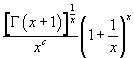is logarithmically completely monotonic in (0,∞) if and only if c≥1 and its reciprocal is logarithmically completely monotonic in (0,∞) if and only if c≤0. The function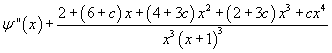is completely monotonic in (0,∞) if and only if c≥1 and its negative is completely monotonic in (0,∞)  if and only if c≤0.

10: Paper Source PDF document

Paper's Title:

An alternative proof of monotonicity for the extended mean values

Author(s):

Chao-Ping Chen and Feng Qi

Department of Applied Mathematics and Informatics, Research Institute of Applied Mathematics,
Henan Polytechnic University, Jiaozuo City, Henan 454000, China

chenchaoping@hpu.edu.cn

Department of Applied Mathematics and Informatics, Research Institute of Applied Mathematics,
Henan Polytechnic University, Jiaozuo City, Henan 454000, China

qifeng@hpu.edu.cn, fengqi618@member.ams.org
Url
: http://rgmia.vu.edu.au/qi.html, http://dami.hpu.edu.cn/qifeng.html

Abstract:

An alternative proof of monotonicity for the extended mean values is given.

10: Paper Source PDF document

Paper's Title:

Equivalence of the Nonsmooth Nonlinear Complementarity Problems to Unconstrained Minimization

Author(s):

M. A. Tawhid and J. L. Goffin

Department of Mathematics and Statistics, School of Advanced Technologies and Mathematics,
Thompson Rivers University,
900 McGill Road, PO Box 3010, Kamloops, BC V2C 5N3
Alexandria University and Egypt Japan University of Science and Technology,
Alexandria-Egypt
mtawhid@tru.ca

Faculty of Management, McGill University,
1001 Sherbrooke Street West, Montreal, Quebec, H3A 1G5
Jean-Louis.Goffin@McGill.ca

Abstract:

This paper deals with nonsmooth nonlinear complementarity problem, where the underlying functions are nonsmooth which admit the H-differentiability but not necessarily locally Lipschitzian or directionally differentiable. We consider a reformulation of the nonlinear complementarity problem as an unconstrained minimization problem. We describe H-differentials of the associated penalized Fischer-Burmeister and Kanzow and Kleinmichel merit functions. We show how, under appropriate P0, semimonotone (E0), P, positive definite, and strictly semimonotone (E) -conditions on an H-differential of f, finding local/global minimum of a merit function (or a `stationary point' of a merit function) leads to a solution of the given nonlinear complementarity problem. Our results not only give new results but also unify/extend various similar results proved for C1.

6: Paper Source PDF document

Paper's Title:

On Some Relations Among the Solutions of the Linear Volterra Integral Equations

Author(s):

Ismet Ozdemir and Faruk Temizer

Inönü Üniversitesi Eğitim Fakültesi,
44280-Malatya,
Turkey

ismet.ozdemir@inonu.edu.tr

omer.temizer@inonu.edu.tr

Abstract:

The sufficient conditions for y1(x)≤ y2(x) were given in  such that ym(x)=fm(x)+∫ax Km(x, t)ym(t)dt,(m=1,2) and x∈ [a, b]. Some properties such as positivity, boundedness and monotonicity of the solution of the linear Volterra integral equation of the form f(t)=1-∫0tK(t-τ)f(τ)dτ=1-K*f, (0≤ t<∞) were obtained, without solving this equation, in [3,4,5,6]. Also, the boundaries for functions f', f'',..., f(n),(n ∈ N) defined on the infinite interval [0, ∞) were found in [7,8].

In this work, for the given equation f(t)=1-K* f and n≥ 2, it is derived that there exist the functions  L2, L3,..., Ln which can be obtained by means of K and some inequalities among the functions f, h2, h3,..., hi for i=2, 3,...., n are satisfied on the infinite interval [0, ∞), where hi is the solution of the equation hi(t)=1-Li* hi and n is a natural number.

5: Paper Source PDF document

Paper's Title:

A note on Inequalities due to Martins, Bennett and Alzer

Author(s):

József Sándor

Babeş-Bolyai University of Cluj, Department of Mathematics and Computer Sciences
Kogălniceanu Nr.1, Cluj-Napoca,
Romania.
jjsandor@hotmail.com
jsandor@member.ams.org

Abstract:

A short history of certain inequalities by Martins, Bennett as well as Alzer, is provided. It is shown that, the inequality of Alzer for negative powers , or Martin's reverse inequality  are due in fact to Alzer . Some related results, as well as a conjecture, are stated.

5: Paper Source PDF document

Paper's Title:

Inequalities of Gamma Function Appearing in Generalizing Probability Sampling Design

Author(s):

Department of Mathematics,
Yarmouk University,
Irbid 21163,
Jordan.

Department of Statistics,
Yarmouk University,
Irbid 21163,
Jordan.

Abstract:

In this paper, we investigate the complete monotonicity of some functions involving gamma function. Using the monotonic properties of these functions, we derived some inequalities involving gamma and beta functions. Such inequalities can be used to generalize different probability distribution functions. Also, they can be used to generalize some statistical designs, e.g., the probability proportional to the size without replacement design.

4: Paper Source PDF document

Paper's Title:

Logarithmically complete monotonicity properties for the gamma functions

Author(s):

Chao-Ping Chen and Feng Qi

Department of Applied Mathematics and Informatics,
Research Institute of Applied Mathematics,
Henan Polytechnic University,
Jiaozuo City, Henan 454010,
China.
chenchaoping@hpu.edu.cn

Department of Applied Mathematics and Informatics,
Research Institute of Applied Mathematics,
Henan Polytechnic University,
Jiaozuo City, Henan 454010,
China.
qifeng@hpu.edu.cn
fengqi618@member.ams.org

Abstract:

Some logarithmically completely monotonic functions involving the gamma functions are presented. As a consequence, some known results are proved and refined.

4: Paper Source PDF document

Paper's Title:

Hyperbolic Barycentric Coordinates

Author(s):

Abraham A. Ungar

Department of Mathematics, North Dakota State University,
Fargo, ND 58105,
USA
Abraham.Ungar@ndsu.edu
URL
: http://math.ndsu.nodak.edu/faculty/ungar/

Abstract:

A powerful and novel way to study Einstein's special theory of relativity and its underlying geometry, the hyperbolic geometry of Bolyai and Lobachevsky, by analogies with classical mechanics and its underlying Euclidean geometry is demonstrated. The demonstration sets the stage for the extension of the notion of barycentric coordinates in Euclidean geometry, first conceived by Möbius in 1827, into hyperbolic geometry. As an example for the application of hyperbolic barycentric coordinates, the hyperbolic midpoint of any hyperbolic segment, and the centroid and orthocenter of any hyperbolic triangle are determined.

3: Paper Source PDF document

Paper's Title:

Reverse of Martin's Inequality

Author(s):

Chao-Ping Chen, Feng Qi, and Sever S. Dragomir

Department of Applied Mathematics and Informatics,
Research Institute of Applied Mathematics,
Henan Polytechnic University,
Jiaozuo City, Henan 454010, China
chenchaoping@sohu.com; chenchaoping@hpu.edu.cn

Department of Applied Mathematics and Informatics,
Research Institute of Applied Mathematics,
Henan Polytechnic University,
Jiaozuo City, Henan 454010, China
qifeng@hpu.edu.cn
Url: http://rgmia.vu.edu.au/qi.html

School of Computer Science and Mathematics,
Victoria University of Technology,
P. O. Box 14428, Melbourne City Mc,
Victoria 8001, Australia
Sever.Dragomir@vu.edu.au
Url: http://rgmia.vu.edu.au/SSDragomirWeb.html

Abstract:

In this paper, it is proved that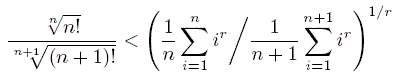for all natural numbers n, and all real r < 0.

3: Paper Source PDF document

Paper's Title:

Generalizations of Hermite-Hadamard's Inequalities for Log-Convex Functions

Author(s):

Ai-Jun Li

School of Mathematics and Informatics, Henan Polytechnic University,
Jiaozuo City, Henan Province,
454010, China.
liaijun72@163.com

Abstract:

In this article, Hermite-Hadamard's inequalities are extended in terms of the weighted power mean and log-convex function. Several refinements, generalizations and related inequalities are obtained.

3: Paper Source PDF document

Paper's Title:

Asymptotic Distribution of Products of Weighted Sums of Dependent Random Variables

Author(s):

Y. Miao and J. F. Li

College of Mathematics and Information Science,
Henan Normal University
Henan, China
yumiao728@yahoo.com.cn

College of Mathematics and Information Science,
Henan Normal University, 453007
Henan, China.
junfen_li@yahoo.com.cn

Abstract:

In this paper we establish the asymptotic distribution of products of weighted sums of dependent positive random variable, which extends the results of Rempała and Wesołowski (2002).

3: Paper Source PDF document

Paper's Title:

Renormalized Solutions for Nonlinear Parabolic Equation with Lower Order Terms

Author(s):

A. Aberqi1, J. Bennouna1, M. Mekkour1 and H. Redwane2

1Université Sidi Mohammed Ben Abdellah,
Département de Mathématiques,
Laboratoire LAMA, Faculté des Sciences Dhar-Mahrez,
B.P 1796 Atlas Fés,
Morocco.

2Faculté des Sciences Juridiques, Economiques et Sociales,
Université Hassan 1, B.P. 784. Settat,
Morocco.

Abstract:

In this paper, we study the existence of renormalized solutions for the nonlinear parabolic problem: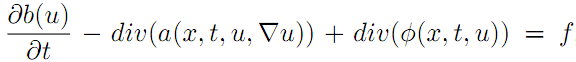,where the right side belongs to L1(Ω×(0,T)) and b(u) is unbounded function of u, the term div(a(x,t,u,u)) is a Leray--Lions operator and the function φ is a nonlinear lower order and satisfy only the growth condition.

3: Paper Source PDF document

Paper's Title:

Stability of an Almost Surjective epsilon-Isometry in The Dual of Real Banach Spaces

Author(s):

Minanur Rohman, Ratno Bagus Edy Wibowo, Marjono

Department of Mathematics, Faculty of Mathematics and Natural Sciences,
Brawijaya University,
Jl. Veteran Malang 65145,
Indonesia.
E-mail: miminanira@gmail.com

Department of Mathematics, Faculty of Mathematics and Natural Sciences,
Brawijaya University,
Jl. Veteran Malang 65145,
Indonesia.
E-mail: rbagus@ub.ac.id

Department of Mathematics, Faculty of Mathematics and Natural Sciences,
Brawijaya University,
Jl. Veteran Malang 65145,
Indonesia.
E-mail: marjono@ub.ac.id

Abstract:

In this paper, we study the stability of epsilon-isometry in the dual of real Banach spaces. We prove that the almost surjective epsilon-isometry mapping is stable in dual of each spaces. The proof uses Gâteaux differentiability space (GDS), weak-star exposed points, norm-attaining operator, and some studies about epsilon-isometry that have been done before.

3: Paper Source PDF document

Paper's Title:

Some properties of k-quasi class Q* operators

Author(s):

Shqipe Lohaj and Valdete Rexhëbeqaj Hamiti

Department of Mathematics,
Faculty of Electrical and Computer Engineering,
University of Prishtina "Hasan Prishtina",
Prishtine 10000,
Kosova.
E-mail: shqipe.lohaj@uni-pr.edu

Department of Mathematics,
Faculty of Electrical and Computer Engineering,
University of Prishtina "Hasan Prishtina",
Prishtine 10000,
Kosova.
E-mail: valdete.rexhebeqaj@uni-pr.edu

Abstract:

In this paper, we give some results of k-quasi class Q* operators. We proved that if T is an invertible operator and N be an operator such that N commutes with T*T, then N is k-quasi class Q* if and only if TNT-1 is of k-quasi class Q*. With example we proved that exist an operator k-quasi class Q* which is quasi nilpotent but it is not quasi hyponormal.

3: Paper Source PDF document

Paper's Title:

Some properties of quasinormal, paranormal and 2-k* paranormal operators

Author(s):

Shqipe Lohaj

Department of Mathematics,
University of Prishtina,
10000, Kosova.
E-mail: shqipe.lohaj@uni-pr.edu

Abstract:

In the beginning of this paper some conditions under which an operator is partial isometry are given. Further, the class of 2-k* paranormal operators is defined and some properties of this class in Hilbert space are shown. It has been proved that an unitarily operator equivalent with an operator of a 2-k* paranormal operator is a 2-k* paranormal operator, and if is a 2-k* paranormal operator, that commutes with an isometric operator, then their product also is a \$2-k^*\$ paranormal operator.

3: Paper Source PDF document

Paper's Title:

Some New Mappings Related to Weighted Mean Inequalities

Author(s):

Xiu-Fen Ma

College of Mathematical and Computer,
No.9 of Xuefu Road, Hechuan District 401520,
Chongqing City,
The People's Republic of China.
E-mail: maxiufen86@163.com

Abstract:

In this paper, we define four mappings related to weighted mean inequalities, investigate their properties, and obtain some new refinements of weighted mean inequalities.

3: Paper Source PDF document

Paper's Title:

Structural and Spectral Properties of k-Quasi Class Q Operators

Author(s):

Valdete Rexhëbeqaj Hamiti and Shqipe Lohaj

Department of Mathematics,
Faculty of Electrical and Computer Engineering,
University of Prishtina "Hasan Prishtina",
Prishtine 10000,
Kosova.
E-mail: valdete.rexhebeqaj@uni-pr.edu

Department of Mathematics,
Faculty of Electrical and Computer Engineering,
University of Prishtina "Hasan Prishtina",
Prishtine 10000,
Kosova.
E-mail: shqipe.lohaj@uni-pr.edu

Abstract:

An operator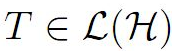is said to be k-quasi class Q if, for all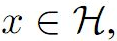where k is a natural number. In this paper, first we will prove some results for the matrix representation of k-quasi class Q operators. Then, we will give the inclusion of approximate point spectrum of k-quasi class Q operators. Also, we will give the equivalence between Aluthge transformation and *-Aluthge transformation of k-quasi class Q operators.

3: Paper Source PDF document

Paper's Title:

On Euler's First Transformation Formula for k-hypergeometric Function

Author(s):

Sungtae Jun and Insuk Kim

General Education Institute,
Konkuk University, Chungju 380-701,
Republic of Korea.
E-mail: sjun@kku.ac.kr

Department of Mathematics Education,
Wonkwang University, Iksan, 570-749,
Republic of Korea.
E-mail: iki@wku.ac.kr

Abstract:

Mubeen et al. obtained Kummer's first transformation for the k-hypergeometric function. The aim of this note is to provide the Euler-type first transformation for the k-hypergeometric function. As a limiting case, we recover the results of Mubeen et al. In addition to this, an alternate and easy derivation of Kummer's first transformation for the k-hypergeometric function is also given.

2: Paper Source PDF document

Paper's Title:

Two Mappings Related to Steffensen's Inequalities

Author(s):

Liang-Cheng Wang

School of Mathematical Science,
Chongqing Institute of Technology,
Xingsheng Lu 4,
Yangjiaping 400050, Chongqing City,
China.
wangliangcheng@163.com

Abstract:

In this paper, we define two mappings closely connected with Steffensen's inequalities, investigate their main properties, give some refinements for Steffensen's inequalities and obtain new inequalities.

2: Paper Source PDF document

Paper's Title:

Oscillations of First Order Linear Delay Difference Equations

Author(s):

G. E. Chatzarakis and I. P. Stavroulakis

Department of Mathematics, University of Ioannina,
451 10, Greece
ipstav@cc.uoi.gr

Abstract:

Consider the first order linear delay difference equation of the form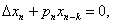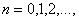where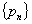is a sequence of nonnegative real numbers, k is a positive integer and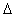denotes the forward difference operator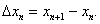New oscillation criteria are established when the well-known oscillation conditions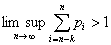and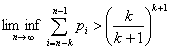are not satisfied. The results obtained essentially improve known results in the literature.

2: Paper Source PDF document

Paper's Title:

Existence Results for Perturbed Fractional Differential Inclusions

Author(s):

Y.-K. Chang

Department of Mathematics,
Lanzhou Jiaotong University, Lanzhou, Gansu 730070, People's
Republic of China
lzchangyk@163.com

Abstract:

This paper is mainly concerned with the following fractional differential inclusions with boundary condition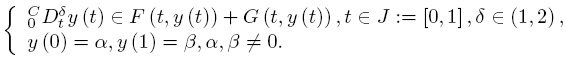A sufficient condition is established for the existence of solutions of the above problem by using a fixed point theorem for multivalued maps due to Dhage. Our result is proved under the mixed generalized Lipschitz and Carathéodory conditions.

2: Paper Source PDF document

Paper's Title:

SEVERAL q-INTEGRAL INEQUALITIES

Author(s):

W. T. Sulaiman

Department of Computer Engineering,
College of Engineering,
University of Mosul,
Iraq
.

Abstract:

In the present paper several q-integral inequalities are presented, some of them are new and others are generalizations of known results.

2: Paper Source PDF document

Paper's Title:

On the Degree of Approximation of Continuous Functions that Pertains to the Sequence-To-Sequence Transformation

Author(s):

Xhevat Z. Krasniqi

University of Prishtina,
Department of Mathematics and Computer Sciences,
5 Mother Teresa Avenue, Prishtinë, 10000,
Republic of Kosovo.

xheki00@hotmail.com

Abstract:

In this paper we prove analogous theorems like Leindler's 3 using the so-called A-transform of the B-transform of the partial sums of Fourier series. In addition, more than two such transforms are introduced and for them analogous results are showed as well.

2: Paper Source PDF document

Paper's Title:

New Refinements of Hölder's Inequality

Author(s):

Xiu-Fen Ma

College of Mathematical and Computer,
No.9 of Xuefu Road, Hechuan District 401520,
Chongqing City,
The People's Republic of China.
E-mail: maxiufen86@163.com

Abstract:

In this paper, we define two mappings, investigate their properties, obtain some new refinements of Hölder's inequality.

2: Paper Source PDF document

Paper's Title:

A Low Order Least-Squares Nonconforming Finite Element Method for Steady Magnetohydrodynamic Equations

Author(s):

Z. Yu, D. Shi and H. Zhu

College of Science,
Zhongyuan University of Technology,
Zhengzhou 450007,
China.
E-mail: 5772@zut.edu.cn

School of Mathematics and Statistics,
Zhengzhou University,
Zhengzhou 450001,
China.
E-mail: shi_dy@126.com

Mathematics Department,
University of Southern Mississippi,
Hattiesburg MS, 39406,
U.S.A
E-mail: huiqing.zhu@usm.edu

Abstract:

A low order least-squares nonconforming finite element (NFE) method is proposed for magnetohydrodynamic equations with EQ1rot element and zero-order Raviart-Thomas element. Based on the above element's typical interpolations properties, the existence and uniqueness of the approximate solutions are proved and the optimal order error estimates for the corresponding variables are derived.

2: Paper Source PDF document

Paper's Title:

Composite Variational-Like Inequalities Given By Weakly Relaxed ζ-Semi-Pseudomonotone Multi-Valued Mapping

Author(s):

Syed Shakaib Irfan, Iqbal Ahmad, Zubair Khan and Preeti Shukla

College of Engineering, Qassim University
Buraidah, Al-Qassim,
Saudi Arabia.
E-mail: shakaib@qec.edu.sa

College of Engineering, Qassim University
Buraidah, Al-Qassim,
Saudi Arabia.
E-mail: iqbal@qec.edu.sa

Department of Mathematics,
Integral University Lucknow,
India.
E-mail: zkhan@iul.ac.in

Department of Mathematics,
Integral University Lucknow,
India.
E-mail: shuklapreeti1991@gmail.com

Abstract:

In this article, we introduce a composite variational-like inequalities with weakly relaxed ζ-pseudomonotone multi-valued maping in reflexive Banach spaces. We obtain existence of solutions to the composite variational-like inequalities with weakly relaxed ζ-pseudomon -otone multi-valued maps in reflexive Banach spaces by using KKM theorem. We have also checked the solvability of the composite variational-like inequalities with weakly relaxed ζ-semi-pseudomonotone multi-valued maps in arbitrary Banach spaces using Kakutani-Fan-Glicksberg fixed point theorem.

2: Paper Source PDF document

Paper's Title:

On The Degree of Approximation of Periodic Functions from Lipschitz and Those from Generalized Lipschitz Classes

Author(s):

Xhevat Z. Krasniqi

Faculty of Education,
University of Prishtina "Hasan Prishtina",
Avenue "Mother Theresa " no. 5, Prishtin
ë 10000,
Republic of Kosovo.
E-mail: xhevat.krasniqi@uni-pr.edu

Abstract:

In this paper we have introduced some new trigonometric polynomials. Using these polynomials, we have proved some theorems which determine the degree of approximation of periodic functions by a product of two special means of their Fourier series and the conjugate Fourier series. Many results proved previously by others are special case of ours.

2: Paper Source PDF document

Paper's Title:

A New Method with Regularization for Solving Split Variational Inequality Problems in Real Hilbert Spaces

Author(s):

Francis Akutsah1 and Ojen Kumar Narain2

1School of Mathematics,
Statistics and Computer Science,
University of KwaZulu-Natal, Durban,
South Africa.
E-mail: 216040405@stu.ukzn.ac.za, akutsah@gmail.com

2School of Mathematics,
Statistics and Computer Science,
University of KwaZulu-Natal, Durban,
South Africa.
E-mail: naraino@ukzn.ac.za

Abstract:

In this paper, we introduce a new inertial extrapolation method with regularization for approximating solutions of split variational inequality problems in the frame work of real Hilbert spaces. We prove that the proposed method converges strongly to a minimum-norm solution of the problem without using the conventional two cases approach. In addition, we present some numerical experiments to show the efficiency and applicability of the proposed method. The results obtained in this paper extend, generalize and improve several results in this direction.

1: Paper Source PDF document

Paper's Title:

Quantitative Estimates for Positive Linear Operators Obtained by Means of Piecewise Linear Functions

Author(s):

Vasile Mihesan

Technical University of Cluj-Napoca,
Department of Mathematics,
Str. C. Daicoviciu 15, Cluj-Napoca,
Romania
Vasile.Mihesan@math.utcluj.ro

Abstract:

In this paper we obtain estimates for the remainder in approximating continuous functions by positive linear operators, using piecewise linear functions.

1: Paper Source PDF document

Paper's Title:

A New Hardy-Hilbert's Type Inequality for Double Series and its Applications

Author(s):

Mingzhe Gao

Department of Mathematics and Computer Science, Normal College Jishou University,
Jishou Hunan, 416000,
People's Republic of China
mingzhegao1940@yahoo.com.cn

Abstract:

In this paper, it is shown that a new Hardy-Hilbert’s type inequality for double series can be established by introducing a parameter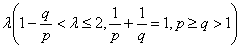and the weight function of the form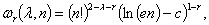where c is Euler constant and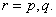And the coefficient is proved to be the best possible. And as the mathematics aesthetics, several important constants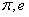and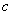appear simultaneously in the coefficient and the weight function when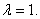In particular, for case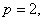some new Hilbert’s type inequalities are obtained. As applications, some extensions of Hardy-Littlewood’s inequality are given.

1: Paper Source PDF document

Paper's Title:

On the Generalized Inverse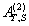over Integral Domains

Author(s):

Yaoming Yu and Guorong Wang

College of Education, Shanghai Normal University
Shanghai 200234
People's Republic of China.
yuyaoming@online.sh.cn
grwang@shnu.edu.cn

Abstract:

In this paper, we study further the generalized inverseof a matrix A over an integral domain. We give firstly some necessary and sufficient conditions for the existence of the generalized inverse, an explicit expression for the elements of the generalized inverseand an explicit expression for the generalized inverse, which reduces to the {1} inverse. Secondly, we verify that the group inverse, the Drazin inverse, the Moore-Penrose inverse and the weighted Moore-Penrose inverse are identical with the generalized inverse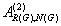for an appropriate matrix G, respectively, and then we unify the conditions for the existence and the expression for the elements of the weighted Moore-Penrose inverse, the Moore-Penrose inverse, the Drazin inverse and the group inverse over an integral domain. Thirdly, as a simple application, we give the relation between some rank equation and the existence of the generalized inverse, and a method to compute the generalized inverse. Finally, we give an example of evaluating the elements ofwithout calculating.

1: Paper Source PDF document

Paper's Title:

Existence of Solutions for Third Order Nonlinear Boundary Value Problems

Author(s):

Yue Hu and Zuodong Yang

School of Mathematics and Computer Science, Nanjing Normal University, Jiangsu Nanjing 210097,
China.
huu3y2@163.com

College of Zhongbei, Nanjing Normal University, Jiangsu Nanjing 210046,
China.
zdyang_jin@263.net
yangzuodong@njnu.edu.cn

Abstract:

In this paper, the existence of solution for a class of third order quasilinear ordinary differential equations with nonlinear boundary value problems

p(u"))'=f(t,u,u',u"), u(0)=A, u'(0)=B, R(u'(1),u"(1))=0

is established. The results are obtained by using upper and lower solution methods.

1: Paper Source PDF document

Paper's Title:

A Geometric Generalization of Busemann-Petty Problem

Author(s):

Liu Rong and Yuan Jun

Shanghai Zhangjiang Group Junior Middle School,
China

Abstract:

The norm defined by Busemann's inequality establishes a class of star body - intersection body. This class of star body plays a key role in the solution of Busemann-Petty problem. In 2003, Giannapoulos  defined a norm for a new class of half-section. Based on this norm, we give a geometric generalization of Busemann-Petty problem, and get its answer as a result

1: Paper Source PDF document

Paper's Title:

Some New Generalizations of Jensen's Inequality with Related Results and Applications

Author(s):

Steven G. From

Department of Mathematics

E-mail: sfrom@unomaha.edu

Abstract:

In this paper, some new generalizations of Jensen's inequality are presented. In particular, upper and lower bounds for the Jensen gap are given and compared analytically and numerically to previously published bounds for both the discrete and continuous Jensen's inequality cases. The new bounds compare favorably to previously proposed bounds. A new method based on a series of locally linear interpolations is given and is the basis for most of the bounds given in this paper. The wide applicability of this method will be demonstrated. As by-products of this method, we shall obtain some new Hermite-Hadamard inequalities for functions which are 3-convex or 3-concave. The new method works to obtain bounds for the Jensen gap for non-convex functions as well, provided one or two derivatives of the nonlinear function are continuous. The mean residual life function of applied probability and reliability theory plays a prominent role in construction of bounds for the Jensen gap. We also present an exact integral representation for the Jensen gap in the continuous case. We briefly discuss some inequalities for other types of convexity, such as convexity in the geometric mean, and briefly discuss applications to reliability theory.

1: Paper Source PDF document

Paper's Title:

Presentation a mathematical model for bone metastases control by using tamoxifen

Author(s):

Maryam Nikbakht, Alireza Fakharzadeh Jahromi and Aghileh Heydari

Department of Mathematics,
Payame Noor University,
P.O.Box 19395-3697, Tehran,
Iran.
.E-mail: maryam_nikbakht@pnu.ac.ir

Department of Mathematics,
Faculty of Basic Science,
Shiraz University of Technology.

Department of Mathematics,
Payame Noor University,
P.O.Box 19395-3697, Tehran,
Iran.
E-mail: a-heidari@pnu.ac.ir

Abstract:

Bone is a common site for metastases (secondary tumor) because of breast and prostate cancer. According to our evaluations the mathematical aspect of the effect of drug in bone metastases has not been studied yet. Hence, this paper suggested a new mathematical model for bone metastases control by using tamoxifen. The proposed model is a system of nonlinear partial differential equations. In this paper our purpose is to present a control model for bone metastases. At end by some numerical simulations, the proposed model is examined by using physician.

1: Paper Source PDF document

Paper's Title:

The Dynamics of an Ebola Epidemic Model with Quarantine of Infectives

Author(s):

Eliab Horub Kweyunga

Department of Mathematics,
Kabale University,
P.O.Box 317, Kabale,
Uganda.

E-mail: hkweyunga@kab.ac.ug

Abstract:

The recurrent outbreaks of ebola in Africa present global health challenges. Ebola is a severe, very fatal disease with case fatality rates of up to 90%. In this paper, a theoretical deterministic model for ebola epidemic with quarantine of infectives is proposed and analyzed. The model exhibits two equilibria; the disease free and endemic equilibrium points. The basic reproduction number, R0, which is the main threshold, is obtained and the stability of the equilibrium points established. Using parameter values drawn from the 2014 West Africa ebola outbreak, a numerical simulation of the model is carried out. It is found that the dynamics of the model are completely determined by R0 and that a quarantine success rate of at least 70% is sufficient to contain the disease outbreak.

1: Paper Source PDF document

Paper's Title:

Inequalities for Discrete F-Divergence Measures: A Survey of Recent Results

Author(s):

Sever S. Dragomir1,2

1Mathematics, School of Engineering & Science
Victoria University, PO Box 14428
Melbourne City, MC 8001,
Australia
E-mail: sever.dragomir@vu.edu.au

2DST-NRF Centre of Excellence in the Mathematical and Statistical Sciences,
School of Computer Science & Applied Mathematics,
University of the Witwatersrand,
Private Bag 3, Johannesburg 2050,
South Africa
URL: http://rgmia.org/dragomir

Abstract:

In this paper we survey some recent results obtained by the author in providing various bounds for the celebrated f-divergence measure for various classes of functions f. Several techniques including inequalities of Jensen and Slater types for convex functions are employed. Bounds in terms of Kullback-Leibler Distance, Hellinger Discrimination and Varation distance are provided. Approximations of the f-divergence measure by the use of the celebrated Ostrowski and Trapezoid inequalities are obtained. More accurate approximation formulae that make use of Taylor's expansion with integral remainder are also surveyed. A comprehensive list of recent papers by several authors related this important concept in information theory is also included as an appendix to the main text.

1: Paper Source PDF document

Paper's Title:

Formulation of Approximate Mathematical Model for Incoming Water to Some Dams on Tigris and Euphrates Rivers Using Spline Function

Author(s):

Nadia M. J. Ibrahem, Heba A. Abd Al-Razak, and Muna M. Mustafa

Mathematics Department,
College of Sciences for Women,
Iraq.

Abstract:

In this paper, we formulate three mathematical models using spline functions, such as linear, quadratic and cubic functions to approximate the mathematical model for incoming water to some dams. We will implement this model on dams of both rivers; dams on the Tigris are Mosul and Amara while dams on the Euphrates are Hadetha and Al-Hindya.

1: Paper Source PDF document

Paper's Title:

Reduced Generalized Combination Synchronization Between Two n-Dimensional Integer-Order Hyperchaotic Systems and One m-Dimensional Fractional-Order Chaotic System

Author(s):

Smail Kaouache, Mohammed Salah Abdelouahab and Rabah Bououden

Laboratory of Mathematics and their interactions,
Abdelhafid Boussouf University Center, Mila.
Algeria
E-mail: smailkaouache@gmail.com, medsalah3@yahoo.fr, rabouden@yahoo.fr

Abstract:

This paper is devoted to investigate the problem of reduced generalized combination synchronization (RGCS) between two n-dimensional integer-order hyperchaotic drive systems and one m-dimensional fractional-order chaotic response system. According to the stability theorem of fractional-order linear system, an active mode controller is proposed to accomplish this end. Moreover, the proposed synchronization scheme is applied to synchronize three different chaotic systems, which are the Danca hyperchaotic system, the modified hyperchaotic Rossler system, and the fractional-order Rabinovich-Fabrikant chaotic system. Finally, numerical results are presented to fit our theoretical analysis.

1: Paper Source PDF document

Paper's Title:

Sharp Inequalities Between Hölder and Stolarsky Means of Two Positive Numbers

Author(s):

M. Bustos Gonzalez and A. I. Stan

The University of Iowa,
Department of Mathematics,
14 MacLean Hall,
Iowa City, Iowa,
USA.
E-mail: margarita-bustosgonzalez@uiowa.edu

The Ohio State University at Marion,
Department of Mathematics,
1465 Mount Vernon Avenue,
Marion, Ohio,
USA.
E-mail: stan.7@osu.edu

Abstract:

Given any index of the Stolarsky means, we find the greatest and least indexes of the H\"older means, such that for any two positive numbers, the Stolarsky mean with the given index is bounded from below and above by the Hölder means with those indexes, of the two positive numbers. Finally, we present a geometric application of this inequality involving the Fermat-Torricelli point of a triangle.

1: Paper Source PDF document

Paper's Title:

Analysis of the Dynamic Response of the Soil-pile Behavioral Model Under Lateral Load

Author(s):

Ibrahima Mbaye, Mamadou Diop, Aliou Sonko and Malick Ba

University of Thies,
Department of Mathematics, Bp 967 Thies,
Senegal.
E-mail: imbaye@univ-thies.sn
aliousonko59@gmail.com
mmalickba@hotmail.fr
URL: https://www.univ-thies.sn

Abstract:

This work aims to extend and improve our previous study on mathematical and numerical analysis of stationary Pasternak model. In this paper a dynamic response of Pasternak model is considered. On the one hand we establish the existence and uniqueness of the solution by using the Lax-Milgram theorem and the spectral theory thus the existence of a Hilbert basis is shown and the spectral decomposition of any solution of the problem can be established and on the other hand the finite element method is used to determinate the numerical results. Furthermore, the influence of soil parameters Gp and Kp on the displacement of the pious is studied numerically at any time tn.

1: Paper Source PDF document

Paper's Title:

Algorithms for Nonlinear Problems Involving Strictly Pseudocontractive Mappings

Author(s):

Mathew Olajiire Aibinu1, Surendra Colin Thakur2, Sibusiso Moyo3

1Institute for Systems Science & KZN E-Skill CoLab,
Durban University of Technology,
Durban 4000,
South Africa.

1DSI-NRF Centre of Excellence in Mathematical and Statistical Sciences (CoE-MaSS),
Johannesburg,
South Africa.
E-mail:
moaibinu@yahoo.com mathewa@dut.ac.za

2 KZN E-Skill CoLab,
Durban University of Technology,
Durban 4000,
South Africa.
E-mail:
thakur@dut.ac.za

3Institute for Systems Science & Office of the DVC Research, Innovation & Engagement Milena Court,
Durban University of Technology,
Durban 4000,
South Africa.
E-mail:
dvcrie@dut.ac.za

Abstract:

The puzzles in approximating a fixed point of nonlinear problems involving the class of strictly pseudocontractive mappings are conquered in this paper through viscosity implicit rules. Using generalized contraction mappings, a new viscosity iterative algorithm which is implicit in nature is proposed and analysed in Banach spaces for the class of strictly pseudocontractive mappings. The computations and analysis which are used in the proposed scheme are easy to follow and this gives rooms for a broad application of the scheme. It is obtained that the proposed iterative algorithm converges strongly to a fixed point of a μ-strictly pseudocontractive mapping which also solves a variational inequality problem. The result is also shown to hold for finite family of strictly pseudocontractive mappings. A numerical example is given to show the skillfulness of the proposed scheme and its implementation.

Search and serve lasted 0 second(s).